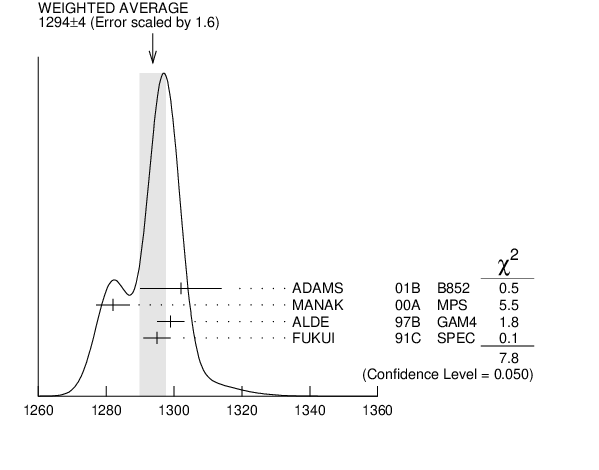# ${{\boldsymbol \eta}{(1295)}}$ MASS INSPIRE search

VALUE (MeV) EVTS DOCUMENT ID TECN  COMMENT
$\bf{ 1294 \pm4}$ OUR AVERAGE  Error includes scale factor of 1.6.
$1302$ $\pm9$ $\pm8$ 20k
 2001 B
B852 18 GeV ${{\mathit \pi}^{-}}$ ${{\mathit p}}$ $\rightarrow$ ${{\mathit K}^{+}}{{\mathit K}^{-}}{{\mathit \pi}^{0}}{{\mathit n}}$
$1282$ $\pm5$ 9082
 2000 A
MPS 18 ${{\mathit \pi}^{-}}$ ${{\mathit p}}$ $\rightarrow$ ${{\mathit \eta}}{{\mathit \pi}^{+}}{{\mathit \pi}^{-}}{{\mathit n}}$
$1299$ $\pm4$ 2100
 1997 B
GAM4 100 ${{\mathit \pi}^{-}}$ ${{\mathit p}}$ $\rightarrow$ ${{\mathit \eta}}{{\mathit \pi}^{0}}{{\mathit \pi}^{0}}{{\mathit n}}$
$1295$ $\pm4$
 1991 C
SPEC 8.95 ${{\mathit \pi}^{-}}$ ${{\mathit p}}$ $\rightarrow$ ${{\mathit \eta}}{{\mathit \pi}^{+}}{{\mathit \pi}^{-}}{{\mathit n}}$
• • • We do not use the following data for averages, fits, limits, etc. • • •
$1264$ $\pm8$ 1
 1990
DM2 ${{\mathit J / \psi}}$ $\rightarrow$ ${{\mathit \gamma}}{{\mathit \eta}}{{\mathit \pi}^{+}}{{\mathit \pi}^{-}}$
$\sim$$1275$
 1979
CNTR 8.4 ${{\mathit \pi}^{-}}$ ${{\mathit p}}$ $\rightarrow$ ${{\mathit n}}{{\mathit \eta}}$2 ${{\mathit \pi}}$
1  PWA analysis of AUGUSTIN 1992 assigns 0${}^{-+}$ quantum numbers to this state rather than 1${}^{++}$ as before.${{\mathit \eta}{(1295)}}$ mass (MeV)
References:
 ADAMS 2001B
PL B516 264 Observation of Pseudoscalar and Axial Vector Resonances in ${{\mathit \pi}^{-}}$ ${{\mathit p}}$ $\rightarrow$ ${{\mathit K}^{+}}{{\mathit K}^{-}}{{\mathit \pi}^{0}}{{\mathit n}}$ at 18 GeV
 MANAK 2000A
PR D62 012003 Partial-Wave Analysis of the ${{\mathit \eta}}{{\mathit \pi}^{+}}{{\mathit \pi}^{-}}$ System Produced in the Reaction ${{\mathit \pi}^{-}}$ ${{\mathit p}}$ $\rightarrow$ ${{\mathit \eta}}{{\mathit \pi}^{+}}{{\mathit \pi}^{-}}{{\mathit n}}$ at 18 ${\mathrm {GeV/}}\mathit c$
 ALDE 1997B
PAN 60 386 Spin Parity Analysis of ${{\mathit \eta}}{{\mathit \pi}^{0}}{{\mathit \pi}^{0}}$ System in ${{\mathit \pi}^{-}}{{\mathit p}}$ Charge Exchange Reaction at 100 ${\mathrm {GeV/}}\mathit c$ by Zemach Method
 FUKUI 1991C
PL B267 293 Study of ${{\mathit \eta}}{{\mathit \pi}^{+}}{{\mathit \pi}^{-}}$ System in the ${{\mathit \pi}^{-}}{{\mathit p}}$ Charge Exchange Reaction at 8.95 ${\mathrm {GeV/}}\mathit c$
 AUGUSTIN 1990
PR D42 10 Radiative Decay of ${{\mathit J / \psi}}$ into ${{\mathit \eta}{(1430)}}$ and Nearby States
 STANTON 1979
PRL 42 346 Evidence for Axial Vector and Pseudoscalar Resonances near 1.275 GeV in ${{\mathit \eta}}{{\mathit \pi}^{+}}{{\mathit \pi}^{-}}$